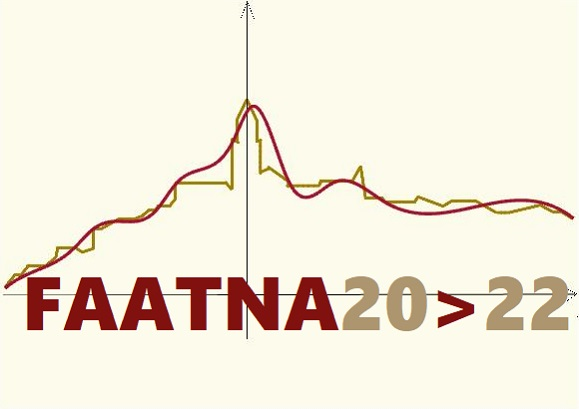# Functional Analysis, Approximation Theory and Numerical Analysis

## Matera, Italy, July 5-8, 2022

Session: Functional Analysis, Approximation Theory and Differential Equations

The Session topics include, but are not limited to:

• new iterative schemes to approximate fixed points of nonlinear mappings, common fixed points of nonlinear mappings or semigroups of nonlinear mappings
• iterative approximations of zeros of accretive-type operators
• iterative approximations of solutions of variational inequalities problems or split feasibility problems and applications
• optimization problems and their algorithmic approaches
• fixed point of nonlinear operators in cone metric spaces with applications and fixed points of nonlinear operators in ordered metric spaces with applications
• applications of Fixed Point Theory to establishing the existence of the solutions of differential equations or integral equations and inclusions
• other theoretical methods for differential and integral equations
• operator equations and inclusions in function spaces

Organizers:

• Vittorio Colao, colao@mat.unical.it
• Luigi Muglia, muglia@mat.unical.it

Talks: